Home | | Biochemistry | The MichaelisŌĆōMenten Approachto Enzyme Kinetics

# The MichaelisŌĆōMenten Approachto Enzyme Kinetics

A particularly useful model for the kinetics of enzyme-catalyzed reactions was devised in 1913 by Leonor Michaelis and Maud Menten.

The MichaelisŌĆōMenten Approachto Enzyme Kinetics

A particularly useful model for the kinetics of enzyme-catalyzed reactions was devised in 1913 by Leonor Michaelis and Maud Menten. It is still the basic model for nonallosteric enzymes and is widely used, even though it has undergone many modi├×cations.

A typical reaction might be the conversion of some substrate, S, to a product, P. The stoichiometric equation for the reaction is

S - > P

The mechanism for an enzyme-catalyzed reaction can be summarized in the formNote the assumption that the product is not converted to substrate to any appreciable extent. In this equation, k1 is the rate constant for the formation of the enzyme-substrate complex, ES, from the enzyme, E, and the substrate, S; k-1 is the rate constant for the reverse reaction, dissociation of the ES complex to free enzyme and substrate; and k2 is the rate constant for the conversion of the ES complex to product P and the subsequent release of product from the enzyme. The enzyme appears explicitly in the mechanism, and the concentrations of both free enzyme, E, and enzyme-substrate complex, ES, therefore, appear in the rate equations. Catalysts characteristically are regenerated at the end of the reaction, and this is true of enzymes.

When we measure the rate (also called the velocity) of an enzymatic reaction at varying substrate concentrations, we see that the rate depends on the substrate concentration, [S]. We measure the initial rate of the reaction (the rate mea-sured immediately after the enzyme and substrate are mixed) so that we can be certain that the product is not converted to substrate to any appreciable extent. This velocity is sometimes written Vinit or V0 to indicate this initial velocity, but it is important to remember that all the calculations involved in enzyme kinetics assume that the velocity measured is the initial velocity. We can graph our results as in Figure 6.8. In the lower region of the curve (at low levels of substrate), the reaction is first order, implying that the velocity, V, depends on substrate concentration [S]. In the upper portion of the curve (at higher levels of substrate), the reaction is zero order; the rate is independent of concentration. The active sites of all of the enzyme molecules are saturated. At infinite substrate concentration, the reaction would proceed at its maximum velocity, written VmaxThe substrate concentration at which the reaction proceeds at one-half its maximum velocity has a special significance. It is given the symbol KM, which can be considered an inverse measure of the affinity of the enzyme for the substrate. The lower the KM, the higher the affinity.

Let us examine the mathematical relationships among the quantities [E], [S], Vmax, and KM. The general mechanism of the enzyme-catalyzed reaction involves binding of the enzyme, E, to the substrate to form a complex, ES, which then forms the product. The rate of formation of the enzyme-substrate complex, ES, iswhere Ōłå[ES]/ Ōłåt means the change in the concentration of the complex, Ōłå [ES], during a given time Ōłåt, and k1 is the rate constant for the formation of the complex.

The ES complex breaks down in two reactions, by returning to enzyme and substrate or by giving rise to product and releasing enzyme. The rate of disap-pearance of complex is the sum of the rates of the two reactions.The negative sign in the term - Ōłå [ES]/ Ōłå t means that the concentration of the complex decreases as the complex breaks down. The term k-1 is the rate constant for the dissociation of complex to regenerate enzyme and substrate, and k2 is the rate constant for the reaction of the complex to give product and enzyme.

Enzymes are capable of processing the substrate very efficiently, and a steadystate is soon reached in which the rate of formation of the enzyme-substratecomplex equals the rate of its breakdown. Very little complex is present, and it turns over rapidly, but its concentration stays the same with time. According to the steady-state theory, then, the rate of formation of the enzyme-substrate complex equals the rate of its breakdown,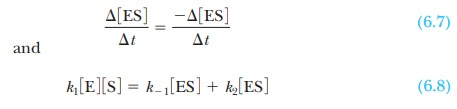To solve for the concentration of the complex, ES, it is necessary to know the concentration of the other species involved in the reaction. The initial concen-tration of substrate is a known experimental condition and does not change sig-nificantly during the initial stages of the reaction. The substrate concentration is much greater than the enzyme concentration. The total concentration of the enzyme, [E]T, is also known, but a large proportion of it may be involved in the complex. The concentration of free enzyme, [E], is the difference between [E]T, the total concentration, and [ES], which can be written as an equation:where KM is called the Michaelis constant. It is now possible to solve Equation 6.11 for the concentration of enzyme-substrate complex:In the initial stages of the reaction, so little product is present that no reverse reaction of product to complex need be considered. Thus the initial rate determined in enzymatic reactions depends on the rate of breakdown of the enzyme-substrate complex into product and enzyme. In the Michaelis-Menten model, the initial rate, V, of the formation of product depends only on the rate of the breakdown of the ES complex,and on the substitution of the expression for [ES] from Equation 6.12,If the substrate concentration is so high that the enzyme is completely saturated with substrate ([ES] = [E]T), the reaction proceeds at its maximum possible rate (Vmax). Substituting [E]T for [ES] in Equation 6.13,The total concentration of enzyme is a constant, which means thatThis expression for Vmax  resembles that for a zero-order reaction given in Equation 6.3:Note that the concentration of substrate, [A], appears in Equation 6.3 rather than the concentration of enzyme, [E], as in Equation 6.15. When the enzyme is saturated with substrate, zero-order kinetics with respect to substrate are observed.

Substituting the expression for Vmax into Equation 6.14 enables us to relate the observed velocity at any substrate concentration to the maximum rate of an enzymatic reaction:Figure 6.8 shows the effect of increasing substrate concentration on the observed rate. In such an experiment, the reaction is run at several substrate concentrations, and the rate is determined by following the disappearance of reactant, or the appearance of product, by way of any convenient method. At low-substrate concentrations, first-order kinetics are observed. At higher sub-strate concentrations (well beyond 10 ├Ś KM), when the enzyme is saturated, the constant reaction rate characteristic of zero-order kinetics is observed.

This constant rate, when the enzyme is saturated with substrate, is the Vmax for the enzyme, a value that can be roughly estimated from the graph. The value of KM can also be estimated from the graph. From Equation 6.16,When experimental conditions are adjusted so that [S] = KM,In other words, when the rate of the reaction is half its maximum value, the substrate concentration is equal to the Michaelis constant (Figure 6.9). This fact is the basis of the graphical determination of KM.

Note that the reaction used to generate the Michaelis-Menten equation was the simplest enzyme equation possible, that with a single substrate going to a single product. Most enzymes catalyze reactions containing two or more substrates. This does not invalidate our equations, however. For enzymes with multiple substrates, the same equations can be used, but only one substrate can be studied at a time. If, for example, we had the enzyme-catalyzed reaction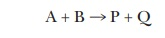we could still use the Michaelis-Menten approach. If we hold A at saturating levels and then vary the amount of B over a broad range, the curve of velocity versus [B] will still be a hyperbola, and we can still calculate the KM for B. Conversely, we could hold the level of B at saturating levels and vary the amount of A to determine the KM for A. There are even enzymes that have two substrates where, if we plot V versus [substrate A], we see the Michaelis-Menten hyperbola, but, if we plot V versus [substrate B], we see the sigmoidal curve shown for aspartate transcarbamoylase in Figure 6.7. Technically the term KM is appropriate only for enzymes that exhibit a hyperbolic curve of velocity versus [substrate].

How do we calculate KM and Vmax from a graph?

The curve that describes the rate of a nonallosteric enzymatic reaction is hyperbolic. It is quite dif├×cult to estimate Vmax because it is an asymptote, and the value is never reached with any ├×nite substrate concentration that we could use in the lab. This, in turn, makes it dif├×cult to determine the KM of the enzyme. It is considerably easier to work with a straight line than a curve. One can transform the equation for a hyperbola (Equation 6.16) into an equation for a straight line by taking the reciprocals of both sides: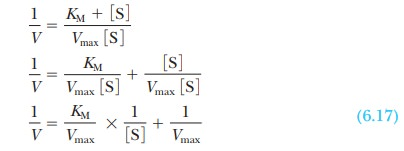The equation now has the form of a straight line, y = mx + b, where 1/V takes the place of the y coordinate and 1/[S] takes the place of the x coordinate. The slope of the line, m, is KM/Vmax, and the y intercept, b, is 1/Vmax. Figure 6.10 presents this information graphically as a LineweaverŌĆōBurk double-reciprocal plot. It is usually easier to draw the best straight line through a set of points thanto estimate the best ├×t of points to a curve. Convenient computer methods exist for drawing the best straight line through a series of experimental points. Such a line can be extrapolated to high values of [S], ones that might be unattainable because of solubility limits or the cost of the substrate. The extrapolated line can be used to obtain Vmax.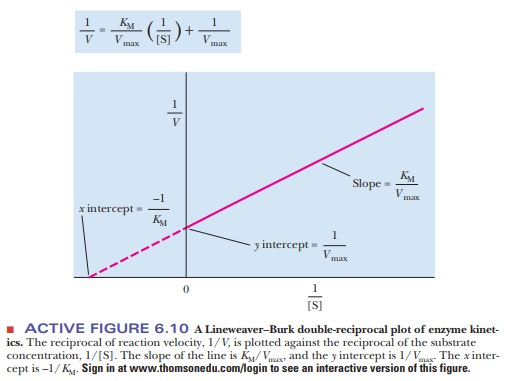What is the significance of KM and Vmax?

We have already seen that when the rate of a reaction, V, is equal to half the maximum rate possible, V = Vmax/2, then KM = [S]. One interpretation of the Michaelis constant, KM, is that it equals the concentration of substrate at which 50% of the enzyme active sites are occupied by substrate. The Michaelis constant has the units of concentration.

Another interpretation of KM  relies on the assumptions of the original Michaelis-Menten model of enzyme kinetics. Recall Equation 6.4:As before, k1 is the rate constant for the formation of the enzyme-substrate complex, ES, from the enzyme and substrate; k-1 is the rate constant for the reverse reaction, dissociation of the ES complex to free enzyme and substrate; and k2 is the rate constant for the formation of product P and the subsequent release of product from the enzyme. Also recall from Equation 6.11 thatConsider the case in which the reaction E + S - > ES takes place more frequently than ES - > E + P. In kinetic terms, this means that the dissociation rate constant k-1is greater than the rate constant for the formation of product, k2. If k-1is much larger than k2 (k-1>>k2), as was originally assumed by Michaelis and Menten, then approximatelyIt is informative to compare the expression for the Michaelis constant with the equilibrium constant expression for the dissociation of the ES complex,The k values are the rate constants, as before. The equilibrium constant expression is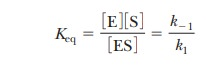This expression is the same as that for KM and makes the point that, when the assumption that k-1>>k2 is valid, KM is simply the dissociation constant for the ES complex. KM is a measure of how tightly the substrate is bound to the enzyme. The greater the value of KM, the less tightly the substrate is bound to the enzyme. Note that in the steady-state approach, k2 is not assumed to be small compared with k-1; therefore, KM is not technically a dissociation constant, even though it is often used to estimate the af├×nity of the enzyme for the substrate.

Vmaxis related to theturnover numberof an enzyme, a quantity equal to thecatalytic constant, k2. This constant is also referred to as kcat or kp: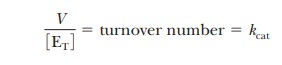The turnover number is the number of moles of substrate that react to form product per mole of enzyme per unit time. This statement assumes that the enzyme is fully saturated with substrate and thus that the reaction is proceeding at the maximum rate. Table 6.2 lists turnover numbers for typical enzymes, where the units are per second.

Turnover numbers are a particularly dramatic illustration of the efficiency of enzymatic catalysis. Catalase is an example of a particularly efficient enzyme. We encountered catalase in its role in converting hydrogen peroxide to water and oxygen. As Table 6.2 indicates, it can transform 40 mil-lion moles of substrate to product every second.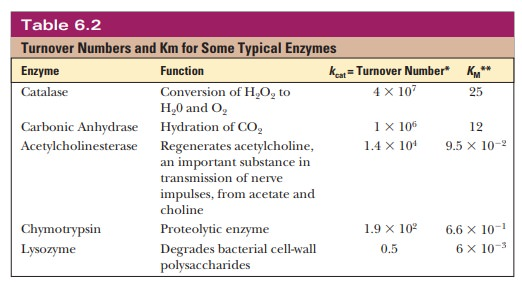Study Material, Lecturing Notes, Assignment, Reference, Wiki description explanation, brief detail
Biochemistry: The Behavior of Proteins: Enzymes : The MichaelisŌĆōMenten Approachto Enzyme Kinetics |